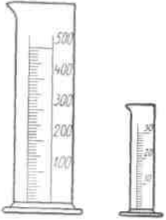# Calculation of the amount of rennet

Calculation of the amount of rennet. By marking the rennet strength, we could also calculate the amount of rennet needed, if we always treated the milk with constant properties at 35 ° C and if the desired clotting period always continued 40 minutes. But this is not the case in practice, because conditions are different here. The properties of milk change, the temperature of the milk varies depending on the requirements, e.g.. within limits 18 do 40°C, the clotting period is also different, e.g.. from 15 minutes to 24 hours. Therefore, according to these changing conditions, the cheesemaker calculates the amount of rennet needed. Before we know, how is he coping in this case, we will know beforehand the law of the operation of rennet. This law establishes it: the amount of rennet is directly proportional to the amount of milk, and inversely proportional to the clotting period, that is, in other words: the more milk we have to season, the more we have to take rennet; the more we take rennet, the shorter the clotting time will be. Here is an example: we have seasoned 852 kg of milk heated to 32 ° C with this amount of rennet, to clot after 30 minutes. We will find the answer by making the following attempt: for the trowel we take from the boiler 100 cm3 of heated milk and season it 1 cm3 of rennet, which we diluted after measuring 5 do 10 times the amount of water. We follow the clotting time with a watch in hand, until the first signs of thickening appear. Let it continue in the given example 30 seconds.

We calculate by proportion, meanwhile, skipping the clotting period: 0,1:852 = 1:x, so x - 8520, that is on 852 we would use kg of milk 8520 cm3 of rennet, if the clotting was only to continue 1/2 minutes, but because it is meant to last 30 minutes, or 60 times longer, We need 60 times less rennet, i.e.. 8520:60 = 142 cm3.

Or you can use the following formula:

where:

M = amount of milk in the boiler, s = clotting time of the sample, m = amount of sample milk, S = time to solidify the milk in the boiler.

You should pay attention to this, to put values ​​of the same order into a formula: M i m w cm3, time either in minutes or in seconds, how more convenient. Having put the given numbers in the above bill, we will get:

(852 X 30) / (0,1 X 1806) = 142 cm³

The purchased liquid rennet should be diluted before the test is performed 5 do 10 fold, powder rennet - 100 fold.

In practice, we usually take a little less rennet, than the test shows, because the calculation inaccuracy resulting from the sample cooling on the trowel should be taken into account, that is, from an inaccurate determination of the point of clotting. Also, it should not be taken as a basis for the sample calculation, which will clot before expiration 15 seconds, because the inaccuracy of the calculation is all the greater, the sooner we get a clot. Better to make a second try and take or more milk, or dilute the rennet accordingly.

If the same tried and tested rennet, we will use it for other products requiring similar conditions, we are not making new attempts, but we decrease or increase the amount of rennet according to the changes made, which usually relate to the amount of milk.

But using natural rennet, freshly prepared every day, we have to make a rehearsal every time. There are also age-old facilities dating back to the times, when there were no clocks yet. Emmental cheese maker, e.g.. calculates the amount of rennet mortar needed as follows, almost countless way: takes from the boiler 6 tablespoons of heated milk and 1 spoon of rennet mortar, mixes on the trowel and counts slowly in seconds. When the sample clots at 15, gives when converting 1000 kg of milk for each number (indeed 1 a second) po 0,1 1 rennet mortar, that is together 1,5 l, and if he could only count 12, would give 1,2 l. The amount of milk is already calculated according to the ratio values, e.g.. at 800 1 one-fifth less. However, this inaccurate calculation cannot be used, if small amounts of milk are seasoned with strong rennet, since in this case the effects of rennet or paint inaccuracies of calculus would be considerable.Glass gauges with a centimeter scale are used to measure a small amount of liquid rennet or to measure the paint.3; larger amounts are measured with a cylindrical gauge of approx 0,5 l with graduation co 5 or 10 cm3.